# Quiz 4: Understanding Money and Its Management

Business

Nominal interest rate is the annual interest rate without taking into consideration the effect of compounding while the effective interest rate is the annual interest rate in which effect of compounding is taken into account during the year. The given interest rate that a bank charge is 1.3% monthly. Here, one need to determine the nominal interest rate and effective interest rate. In order to determine the annual nominal interest rate, follow the below calculations: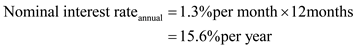Hence, the nominal interest rate is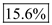. Now in order to determine the effective interest rate, use the following formula: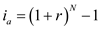Where I a refers to the annual interest rate (which is unknown in this case), i refers to the periodical interest rate for the time period reviewed, and N refers to the number of terms that the money is for. The annual interest rate calculated above is 15.6% and N is 12. Substitute these values and solve for nominal interest rate as follows: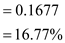Hence, the effective interest rate is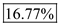.

The nominal interest rate refers to the annual interest rate without taking into consideration the effect of compounding while the effective interest rate is the annual interest rate in which effect of compounding is taken into account during the year. Person A expects the average monthly balance of \$300 with the saving account of 6%. The data for each bank term is given. (a) It is required to find the effective annual interest rate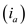for each card. For Bank A: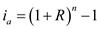Where R is the finance charge of each bank and n is the total number of months. It can be solved as done below: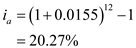For Bank B:(b) It is needed to find the bank which the person A should choose. For this, it is needed to find the present worth of both the banks. The interest rate is given to be 6% compounded daily. Now, it is needed to find the per day interest rate which can be calculated as done below:Now, it is needed to find the effective interest rate per payment period.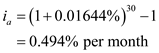It was also expected that remaining balance of \$300 had been paid off for the 30 years. The finance charge is paid on the amount \$300 for both the banks. For Bank A,For Bank B:The present worth of Bank A is calculated as done below: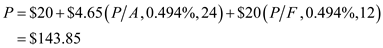The present worth of Bank B is calculated as done below: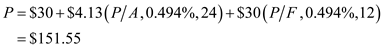The present worth of Bank A is less. Hence, the person A will choose Bank A. (c) It is required to find the time required to pay off the total debt. It can be calculated with the appropriate formula in the spreadsheet given below: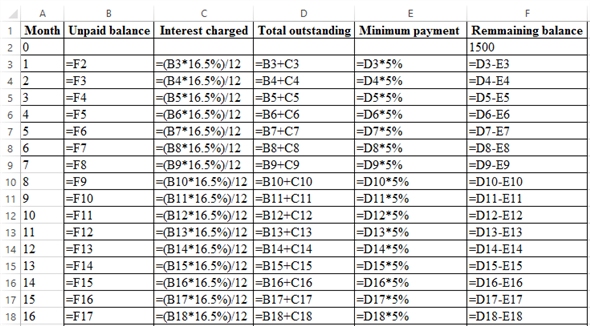The corresponding values are: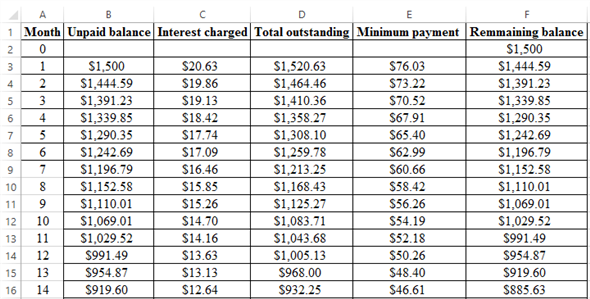Hence, the person A requires 59 months to pay off the debt as the remaining balance at the year 59 is zero.

Nominal interest rate is the annual interest rate without taking into consideration the effect of compounding while the effective interest rate is the annual interest rate in which effect of compounding is taken into account during the year. The given interest rate that charged on credit card is 17.85% compounded on monthly basis. a. Here, one need to determine the monthly interest rate. In order to determine the monthly interest rate, follow the below calculations: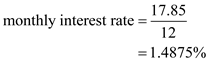Hence, the monthly interest rate is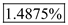. Now in order to determine the effective interest rate, use the following formula:Where I a refers to the annual interest rate (which is unknown in this case), i refers to the periodical interest rate for the time period reviewed, and N refers to the number of terms that the money is for. The annual interest rate calculated above is 15.6% and N is 12. Substitute these values and solve for nominal interest rate as follows: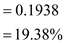Hence, the effective interest rate is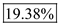. b. The total balance amount is to determine if the two-month payment of \$2,500 of each month is skipped. The balance amount is calculated as follows:Hence, the balance amount is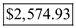.